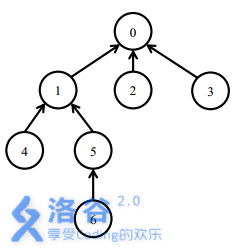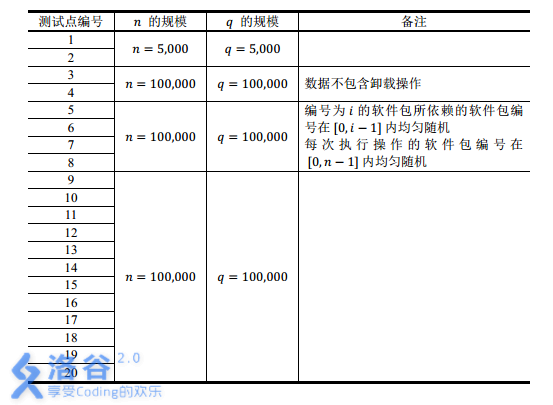# 树链剖分 | 线段树 | 树链剖分：学习笔记

## 树链剖分

• 将树上两点 $(u,\;v)$ 之间的所有点权值加上 $\delta$

• 询问 $\sum^{v}_{i=u}w[i]$，表示从 $u$ 到 $v$ 的唯一路径上的所有点权值之和

• 询问 $max\lbrace w[i]\;\;\; i\in\lbrace u,\;v \rbrace \rbrace$，表示询问 $u$ 到 $v$ 的唯一路径上的点权最大（最小亦同）

## 概念

1. 重儿子：结点的重儿子定义为结点的直接儿子中，子树大小最大的那一个结点
• 对于儿子们的子树大小相等的情况，随意取一个作为重儿子即可，不会造成影响
2. 轻儿子：除去重儿子之外的其他直接儿子
3. 重边：结点与他的重儿子之间的边
4. 轻边：结点与他的轻儿子之间的边
5. 深度：根结点到这个结点的结点个数（包括根结点和他自己）

1. 子树大小（包括子树的根结点）
2. TOP 值：记录的是当前结点所在重边的起点（轻儿子的 TOP 值是他自己的编号）

## 计算和预处理

### 第一次 DFS：计算前面 6 个值

void DFS(int u){
siz[u]=1; int maxsize=0;
if(!dep[to[i]]){
dep[to[i]]=dep[u]+1;
DFS(to[i]);
siz[u]+=siz[to[i]]; fa[to[i]]=u;
if(siz[to[i]]>maxsize){
maxsize=siz[to[i]];
Xson[u]=to[i];
}
}
}


### 第二次 DFS：计算 TOP 值

void DFS(int u,int TOP){
id[u]=++ids;
top[u]=TOP;
if(siz[u]==1) return;
DFS(Xson[u],TOP);// 优先走重儿子
if(to[i]!=Xson[u] && fa[u]!=to[i])
DFS(to[i],to[i]);
}


### 与线段树的配合

graph TD
1---2;2===4;2---5;
1===3;3---6;3===7;7===8


graph TD
d1(("1,1"));d3(("3,2"));d7(("7,3"))
d8(("8,4"));d6(("6,5"));d2(("2,6"))
d4(("4,7"));d5(("5,8"))
d1---d2;d1===d3;
d2---d5;d2===d4;d3===d7;
d3---d6; d7===d8


## 修改操作

### 修改 $(u,v)$ 绝对路径上的点权值

inline void pathadd(int x,int y,int dt){
// 将 x->y 最短路径上的点权值都加上 d
while(top[x]!=top[y]){
if(dep[top[x]]<dep[top[y]]) swap(x,y);
SST.updata(1,1,n,id[top[x]],id[x],dt);
x=fa[top[x]];
}
if(dep[x]<dep[y]) swap(x,y);
SST.updata(1,1,n,id[y],id[x],dt);
}


### 修改 $u$ 的子树的结点权值

updata(1,1,n,id[x],id[x]+siz[x]-1,z);


## 询问操作

### 询问 $(u,v)$ 绝对路径上的点权值和

SST 在代码中表示用结构体定义的线段树，支持区间修改 & 区间求和

long long pathsum(int x,int y){
// 返回 x-> y 的最短路径上的点权值和
long long ret=0;
while(top[x]!=top[y]){
if(dep[top[x]]<dep[top[y]]) swap(x,y);
ret+=SST.SegSum(1,1,n,id[top[x]],id[x]);
x=fa[top[x]];
}
if(dep[x]<dep[y]) swap(x,y);
ret+=SST.SegSum(1,1,n,id[y],id[x]);
return ret
}


### 修改 $u$ 的子树的结点权值和

SST 在代码中表示用结构体定义的线段树，支持区间修改 & 区间求和（再说一遍）

SST.SegSum(1,1,n,id[x],id[x]+siz[x]-1)


## 例题1：洛谷P3384【模板】树链剖分

### Sample Input

5 5 2 24
7 3 7 8 0
1 2
1 5
3 1
4 1
3 4 2
3 2 2
4 5
1 5 1 3
2 1 3


### Sample Output

2
21


### Hint

30% $\leq 10$ $\leq 10$
70% $\leq 10^3$ $\leq 10^3$
100% $\leq 10^5$ $\leq 10^5$

### Codes

// 洛谷 P3384 树链剖分 模板
#include <cstdio>
#include <cstring>
#include <algorithm>
#include <queue>
#include <vector>
#define maxn 100001
#define maxm maxn*2
using namespace std;
int n,m,root,mod,ids;
struct SegSumTree{
typedef long long ll;
struct SegSumTreeNode{
ll V; int LC,RC;
ll TAG;
#define val(x) T[x].V
#define lc(x) T[x].LC
#define rc(x) T[x].RC
#define tag(x) T[x].TAG
#define mid ((L+R)>>1)
}T[maxn<<2];
int nodes;
int Tr[maxn];
template<typename t>inline void fcin(t &x){
int sign=1; x=0; char op=getchar();
while(op<'0'||op>'9'){if(op=='-') sign=-1;op=getchar();}
while(op>='0'&&op<='9'){x=x*10+(op-48);op=getchar();}
x*=sign;
}
inline void pushup(int k){val(k)=val(lc(k))+val(rc(k));}
inline void Spr(int k,int L,int R,int d){
tag(k)+=d; val(k)+=(R-L+1)*(ll)d;
tag(k)%=mod; val(k)%=mod;
}
inline void pushdown(int k,int L,int R){
if(tag(k)==0) return;
Spr(lc(k),L,mid,tag(k));
Spr(rc(k),mid+1,R,tag(k));
tag(k)=0;
}
inline void BuildTree(int N){
nodes=0;build(1,N);
}
int build(int L,int R){
int now=++nodes;
if(L==R) {val(now)=Tr[L];return now;}
lc(now)=build(L,mid);
rc(now)=build(mid+1,R);
pushup(now);
return now;
}
void updata(int k,int L,int R,int x,int y,int d){
if(x<=L && R<=y) return Spr(k,L,R,d);
pushdown(k,L,R);
if(mid>=x) updata(lc(k),L,mid,x,y,d);
if(mid<y) updata(rc(k),mid+1,R,x,y,d);
pushup(k);
}
ll SegSum(int k,int L,int R,int x,int y){
if(x<=L && R<=y) return val(k);
pushdown(k,L,R); ll sum=0;
if(mid>=x) sum+=SegSum(lc(k),L,mid,x,y),sum%=mod;
if(mid<y) sum+=SegSum(rc(k),mid+1,R,x,y),sum%=mod;
return sum;
}
};
SegSumTree SST;
int w[maxn],Xson[maxn],dep[maxn],fa[maxn],top[maxn],tot;// 注意这里是点权值，不是边权值
void DFS(int u){
siz[u]=1; int maxsize=0;
if(!dep[to[i]]){
dep[to[i]]=dep[u]+1; DFS(to[i]);
siz[u]+=siz[to[i]]; fa[to[i]]=u;
if(siz[to[i]]>maxsize){
maxsize=siz[to[i]];
Xson[u]=to[i];
}
}
}
void DFS(int u,int TOP){
id[u]=++ids; SST.Tr[ids]=w[u];
top[u]=TOP;
if(siz[u]==1) return;
DFS(Xson[u],TOP);// 优先走重儿子
if(to[i]!=Xson[u] && fa[u]!=to[i])
DFS(to[i],to[i]);
}
}
inline void pathadd(int x,int y,int dt){
// 将 x->y 最短路径上的点权值都加上 d
while(top[x]!=top[y]){
if(dep[top[x]]<dep[top[y]]) swap(x,y);
SST.updata(1,1,n,id[top[x]],id[x],dt);
x=fa[top[x]];
}
if(dep[x]<dep[y]) swap(x,y);
SST.updata(1,1,n,id[y],id[x],dt);
}
long long pathsum(int x,int y){
// 返回 x-> y 的最短路径上的点权值和
long long ret=0;
while(top[x]!=top[y]){
if(dep[top[x]]<dep[top[y]]) swap(x,y);
ret+=SST.SegSum(1,1,n,id[top[x]],id[x]);
ret%=mod; x=fa[top[x]];
}
if(dep[x]<dep[y]) swap(x,y);
ret+=SST.SegSum(1,1,n,id[y],id[x]);
return ret%mod;
}
int main(){
#ifndef ONLINE_JUDGE
freopen("testin.txt","r",stdin);
freopen("testout.txt","w",stdout);
#endif
SST.fcin(n); SST.fcin(m);
SST.fcin(root); SST.fcin(mod);
int x,y,z,op;
for(int i=1;i<=n;i++) SST.fcin(w[i]);
for(int i=1;i<=n-1;i++){
SST.fcin(x);SST.fcin(y);
} dep[root]=1; DFS(root);
DFS(root,root); SST.BuildTree(ids);
for(int i=1;i<=m;i++){
SST.fcin(op);
switch(op){
case 1: SST.fcin(x);SST.fcin(y);SST.fcin(z);
break;
case 2: SST.fcin(x);SST.fcin(y);
printf("%lld\n",pathsum(x,y));
break;
case 3: SST.fcin(x);SST.fcin(z);
SST.updata(1,1,n,id[x],id[x]+siz[x]-1,z);
break;
case 4: SST.fcin(x);
printf("%lld\n",SST.SegSum(1,1,n,id[x],id[x]+siz[x]-1));
break;
}
}
return 0;
}


### Extra

1. 洛谷P3833 SHOI2012 魔法树

2. 洛谷P2590 ZJOI2008 树的统计
3. P2590 ZJOI2008 树的统计
• 这个题把区间最大线段树和区间求和线段树 rua 在了一起，但是本质没有变化，按照模板格式直接套进去就完事

## 例题2：洛谷P2146 NOI2015软件包管理器

### Description

Linux用户和OSX用户一定对软件包管理器不会陌生。通过软件包管理器，你可以通过一行命令安装某一个软件包，然后软件包管理器会帮助你从软件源下载软件包，同时自动解决所有的依赖（即下载安装这个软件包的安装所依赖的其它软件包），完成所有的配置。Debian/Ubuntu使用的apt-get，Fedora/CentOS使用的yum，以及OSX下可用的homebrew都是优秀的软件包管理器。

### Input

install x：表示安装软件包x

uninstall x：表示卸载软件包x

### Sample Input

7
0 0 0 1 1 5
5
install 5
install 6
uninstall 1
install 4
uninstall 0


### Sample Output

3
1
3
2
3


### Hint

【样例说明】【数据范围】### 分析

#include <cstdio>
#include <cstring>
#include <queue>
#include <iostream>
#include <vector>
#include <cmath>
#include <algorithm>
#define maxn 100001
#define maxm maxn*2
using namespace std;
typedef long long ll;
struct SegCovTree{
struct SegCovTreeNode{
int VAL,LC,RC,TAG;
#define val(x) T[x].VAL
#define lc(x) T[x].LC
#define rc(x) T[x].RC
#define tag(x) T[x].TAG
#define mid ((L+R)>>1)
}T[maxn<<1]; int nodes;
template<typename t>inline void fcin(t &x){
int sign=1; x=0; char op=getchar();
while(op<'0'||op>'9'){if(op=='-') sign=-1;op=getchar();}
while(op>='0'&&op<='9'){x=x*10+(op-48);op=getchar();}
x*=sign;
}
inline void pushup(int k){
val(k)=val(lc(k))+val(rc(k));
}
inline void pushdown(int k,int L,int R){
if(tag(k)==-1) return;
val(lc(k))=tag(k)*(mid-L+1);
val(rc(k))=tag(k)*(R-mid);
tag(lc(k))=tag(rc(k))=tag(k);
tag(k)=-1;
}
inline void BuildTree(int N){
nodes=0; build(1,N);
}
int build(int L,int R){
int now=++nodes; tag(now)=-1;
if(L==R){ val(now)=0;return now; }
lc(now)=build(L,mid);
rc(now)=build(mid+1,R);
pushup(now);
return now;
}
void updata(int k,int L,int R,int x,int y,int d){
// d 为要覆盖的值
if(x<=L && R<=y){
val(k)=d*(R-L+1); tag(k)=d;
return;
} pushdown(k,L,R);
if(x<=mid) updata(lc(k),L,mid,x,y,d);
if(y>mid) updata(rc(k),mid+1,R,x,y,d);
pushup(k);
}
int SegSum(int k,int L,int R,int x,int y){
if(x<=L && R<=y) return val(k);
pushdown(k,L,R); int sum=0;
if(x<=mid) sum+=SegSum(lc(k),L,mid,x,y);
if(y>mid) sum+=SegSum(rc(k),mid+1,R,x,y);
return sum;
}
}; SegCovTree SCT;
int n,q,ids,root;
int w[maxn],Xson[maxn],dep[maxn],fa[maxn],top[maxn],tot;
}
void DFS(int u){
siz[u]=1; int maxsize=0;
if(!dep[to[i]]){
dep[to[i]]=dep[u]+1;
DFS(to[i]);
siz[u]+=siz[to[i]]; fa[to[i]]=u;
if(siz[to[i]]>maxsize){
maxsize=siz[to[i]];
Xson[u]=to[i];
}
}
}
void DFS(int u,int TOP){
id[u]=++ids;
top[u]=TOP;
if(siz[u]==1) return;
DFS(Xson[u],TOP);// 优先走重儿子
if(to[i]!=Xson[u] && fa[u]!=to[i])
DFS(to[i],to[i]);
}
inline void pathcov(int x,int y,int dt){
while(top[x]!=top[y]){
if(dep[top[x]]<dep[top[y]]) swap(x,y);
SCT.updata(1,1,n,id[top[x]],id[x],dt);
x=fa[top[x]];
}
if(dep[x]<dep[y]) swap(x,y);
SCT.updata(1,1,n,id[y],id[x],dt);
}
int pathsum(int x,int y){
// 返回 x-> y 的最短路径上的点权值和
int ret=0;
while(top[x]!=top[y]){
if(dep[top[x]]<dep[top[y]]) swap(x,y);
ret+=SCT.SegSum(1,1,n,id[top[x]],id[x]);
x=fa[top[x]];
}
if(dep[x]<dep[y]) swap(x,y);
ret+=SCT.SegSum(1,1,n,id[y],id[x]);
return ret;
}
int main(){
#ifndef ONLINE_JUDGE
freopen("testin.txt","r",stdin);
freopen("testout.txt","w",stdout);
#endif
SCT.fcin(n); root=1;
int a,b; char op;
for(int i=2;i<=n;i++){
SCT.fcin(a);a++;
} dep[root]=1; int TMP;
DFS(root);DFS(root,root);
SCT.BuildTree(n); SCT.fcin(q);
while(q--){
scanf("%s",op);
SCT.fcin(a);a++;
if(op=='i'){
TMP=pathsum(root,a);
pathcov(root,a,1);
printf("%d\n",pathsum(root,a)-TMP);
}else{
printf("%d\n",SCT.SegSum(1,1,n,id[a],id[a]+siz[a]-1));
SCT.updata(1,1,n,id[a],id[a]+siz[a]-1,0);
}
}
return 0;
}


## 感想

dep[root]=1;